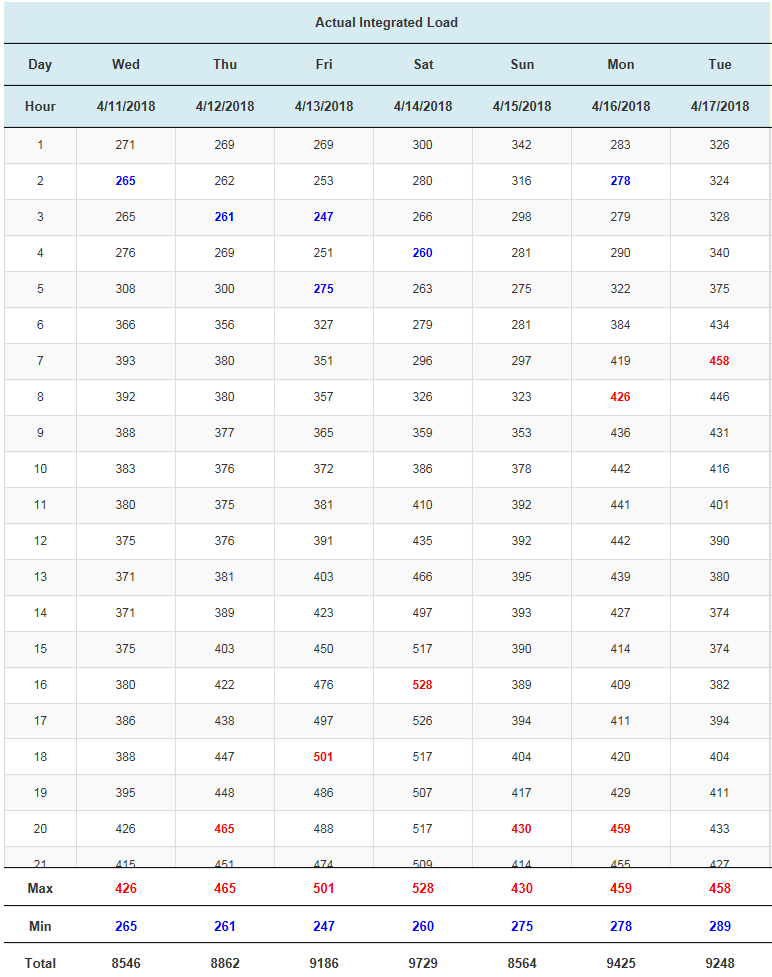# Add css class to cell holding min & max value in a column is adding class to wrong table cell

I am using the footer callback to calculate the min, max and totals (refer to https://datatables.net/forums/discussion/56412). in the same footer call i am also trying to add the class to the table cell where the value is located. It partially works but sometimes it will add the class to a table cell in the wrong column. I have tried to write this code many different ways but always find that if the value appears in numerous columns/rows the row/column where it first appears is the cell that gets the css applied. Can someone help? Heres my code:

``````        "footerCallback": function (row, data, start, end, display) {
var api = this.api();
nb_cols = api.columns().nodes().length;
var j = 1;
while (j < nb_cols) {
var totVal = api
.column(j, { page: 'current' })
.data()
.reduce(function (a, b) {
return Number(a) + Number(b);
}, 0);

var maxVal = api
.column(j, { page: 'current' })
.data()
.sort(function (a, b) { return a - b; })
.reverse();

var minVal = api
.column(j, { page: 'current' })
.data()
.sort(function (a, b) { return a - b; });

if (typeof maxVal !== "undefined") {

var maxCol = api.column(j).nodes().cell(":contains(" + maxVal + ")").column;
var maxRow = api.column(j).nodes().cell(":contains(" + maxVal + ")").row;

var minCol = api.column(j).nodes().cell(":contains(" + minVal + ")").column;
var minRow = api.column(j).nodes().cell(":contains(" + minVal + ")").row;

// Update footer
\$('#amax' + j).html(maxVal);
\$('#amin' + j).html(minVal);
\$('#atot' + j).html(totVal);
}

j++;
}
}
``````

I've also tried this with the same result (I'm wondering if the "contains" is the problem).

``````                    \$(api.column(j).cell(":contains(" + maxVal + ")").node()).addClass('cellmax');
``````## Replies

Hi @johnsonj ,

We're happy to take a look, but as per the forum rules, please link to a test case - a test case that replicates the issue will ensure you'll get a quick and accurate response. Information on how to create a test case (if you aren't able to link to the page you are working on) is available here.

Cheers,

Colin

• Sorry. I created the test case here: http://live.datatables.net/bepiloso/1/edit

Hi @johnsonj ,

Here it is, working I believe as expected. The problem was that

``````api.column(j).cell(":contains(" + maxVal + ")")
``````

wasn't working how you'd expect - that wouldn't return just the cells in that column since `column()` returns a full API instance, not specific to that column - instead the `cell()` would return cells across all columns.

I changed the code to iterate through the column to find the min and max values.

Cheers,

Colin

• Thanks Colin! It works great! I went back and forth about looping through the rows the way you did but thought there must be a way to loop through the column cells. So based on this solution, am I correct to assume that there is no way to loop through the cells in a column with column/row coordinates? I understand that its not exactly necessary to code it. It's just a bit unexpected that the API allows you to specify row/column coordinates but not column/row coordinates.

You could use `cells().every()`, with `null` for the row selector, as in this example here. I'm old school - I like `for` loops